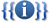## FANDOM

1,085 Pages

 [history] [purge]Template documentation

## Syntax

 Basic - Most Common (Author) ```{{cite web | url = | title = | author = | date = | work = | publisher = | accessdate = }}``` Basic - Most Common (Last, First) ```{{cite web | url = | title = | last = | first = | date = | work = | publisher = | accessdate = }}``` Full Paremter - Author ```{{cite web | url = | title = | author1 = | authorlink1 = | author2 = | authorlink2 = | date = | month = | year = | work = | publisher = | location = | page = | pages = | at = | language = | trans_title = | type = | format = | arxiv = | asin = | bibcode = | doi = | doibroken = | isbn = | issn = | jfm = | jstor = | lccn = | mr = | oclc = | ol = | osti = | pmc = | pmid = | rfc = | ssrn = | zbl = | id = | archiveurl = | archivedate = | deadurl = | accessdate = | quote = | ref = | separator = | postscript = }}``` Full Parameter - Last, First ```{{cite web | url = | title = | last1 = | first1 = | authorlink1 = | last2 = | first2 = | authorlink2 = | date = | month = | year = | work = | publisher = | location = | page = | pages = | at = | language = | trans_title = | type = | format = | arxiv = | asin = | bibcode = | doi = | doibroken = | isbn = | issn = | jfm = | jstor = | lccn = | mr = | oclc = | ol = | osti = | pmc = | pmid = | rfc = | ssrn = | zbl = | id = | archiveurl = | archivedate = | deadurl = | accessdate = | quote = | ref = | separator = | postscript = }}```
Community content is available under CC-BY-SA unless otherwise noted.